# Speed-Time Graphs

GCSELevel 4-5Edexcel iGCSE

## Speed-Time Graphs

Speed-time graphs (or velocity-time graphs) graph an object’s movement over time.

Time is plotted along the $x$-axis and is measured in a unit of time, usually seconds.

Speed is plotted along the $y$-axis and is usually measured in meters per second ($\text{m} / \text{s}$ or $\text{ms}^{-1}$)

A unique feature about speed-time graphs is how much information we can attain from them

## Speed-Time Graph Basics

We may discern from a speed time graph a lot of information about the movement of the object.

The gradient of the graph is the objects acceleration.

A negative gradient means an object is decelerating

flat line means the object is moving with a constant speed.

The area under the curve is the distance the object has travelled.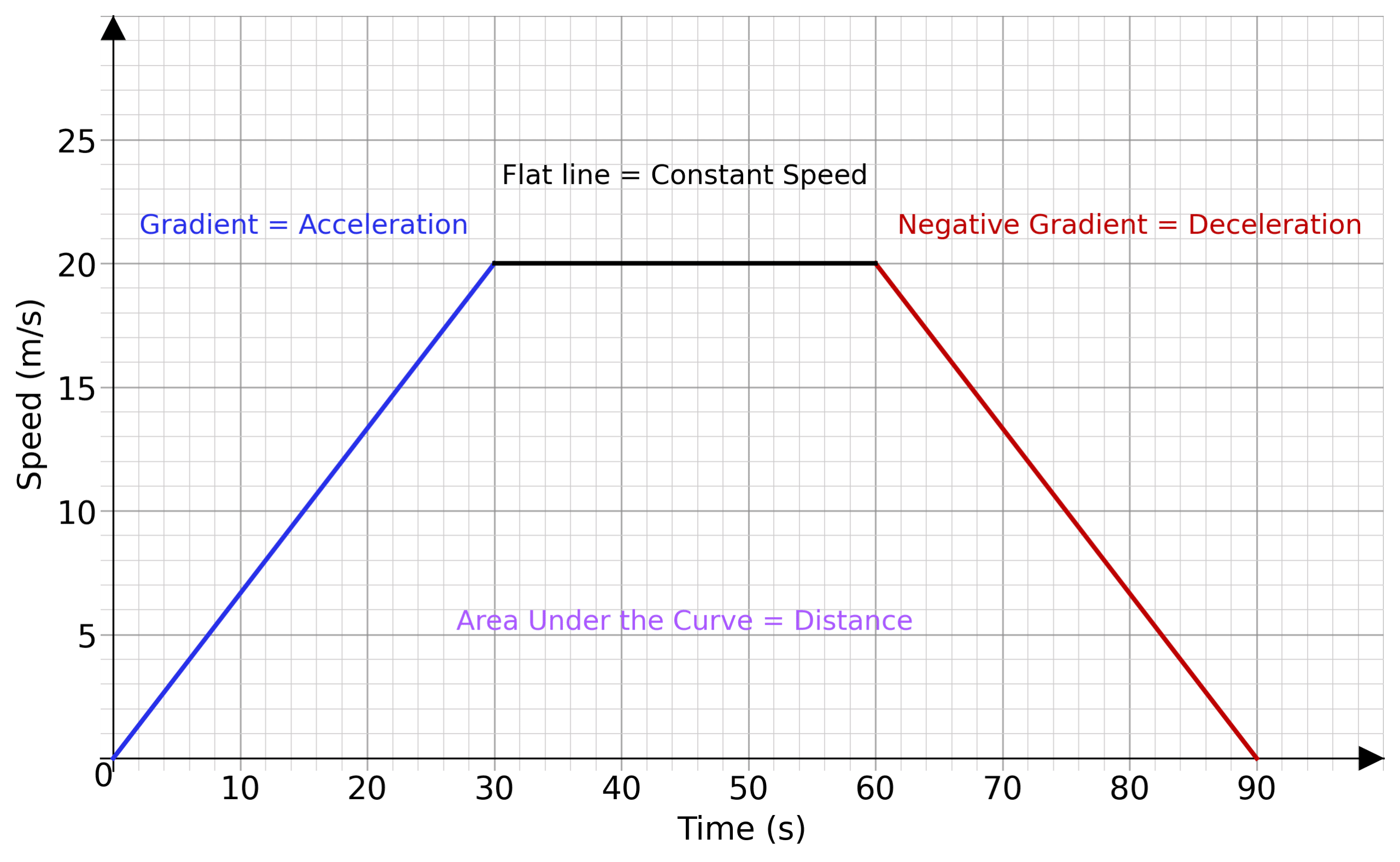Level 4-5GCSEEdexcel iGCSE

## Describing Speed-Time Graphs

We need to be able to describe the speed-time graph. One way to fully describe a speed-time graph is to break up the journey into parts.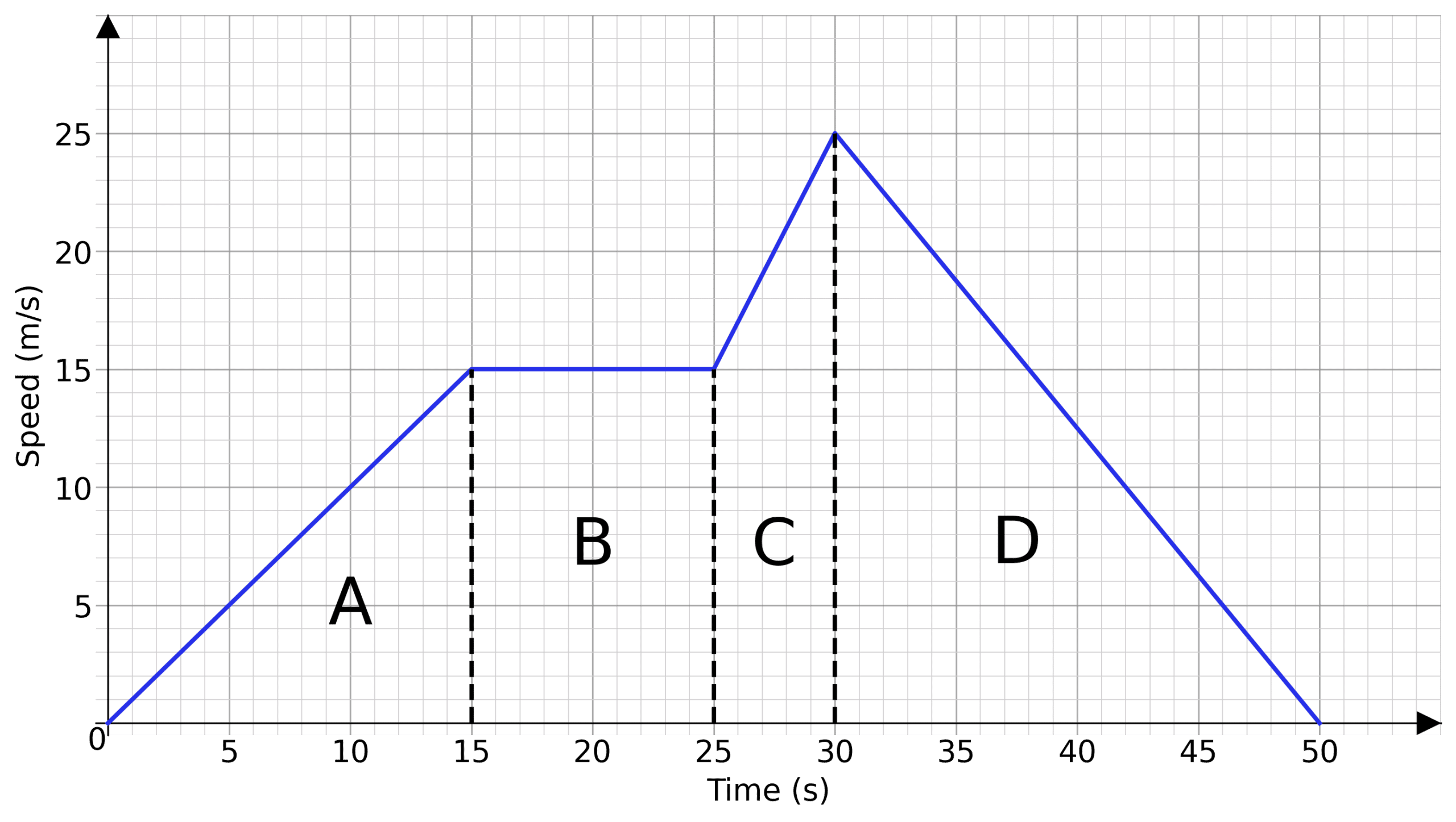Here the graph has been broken up into four sections.  $A$, $B$, $C$ and $D$. Describing them individually is easier than describing the graph in one go. In this example

$A$: The object accelerates from $0 \text{ ms}^{-1}$ to $15 \text{ ms}^{-1}$ over $15$ seconds.

$B$: Then the object travels at a constant speed of $15\text{ ms}^{-1}$ for $10$ seconds.

$C$: The object then accelerates again from $15\text{ ms}^{-1}$ to $25\text{ ms}^{-1}$ in $5$ seconds.

$D$The object then decelerates from $25\text{ ms}^{-1}$ to stationary in $20$ seconds.

Level 4-5GCSEEdexcel iGCSE

## Calculating Acceleration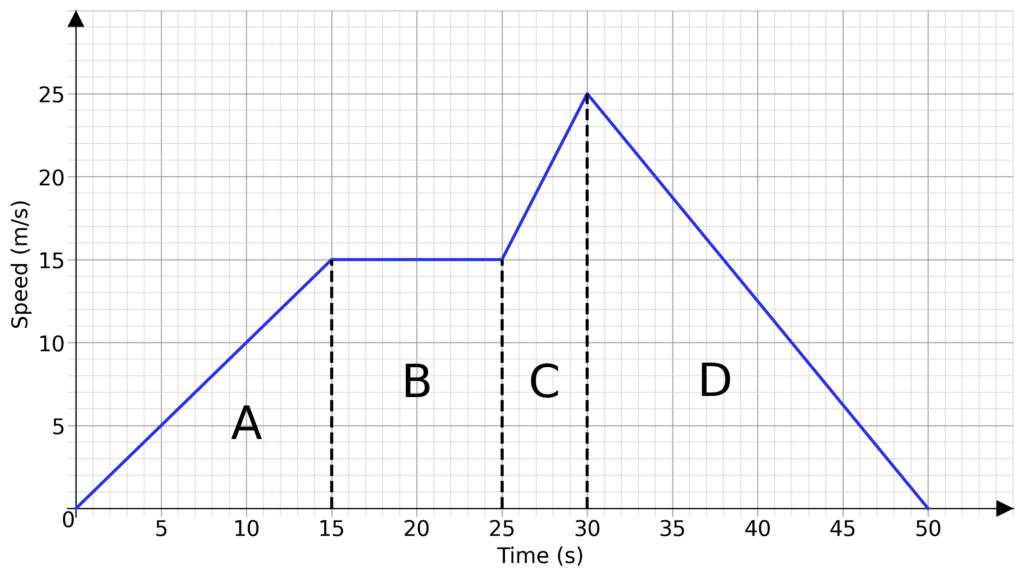$\text{Acceleration }$$=$$\text{ The Gradient of the Line}$

Using the previous example, we shall still divide the graph and find the gradient for each section.

Remember that

$\text{Gradient} = \dfrac{\text{Difference in } y}{\text{Difference in } x}$

given that we can find the acceleration, which is measured in meters per second$^{2}$ or $\text{ms}^{-2}$.

$A$: The gradient is then the difference in speed divided by the difference in time, which here gives us

$\text{Acceleration}= \dfrac{15-0}{15-0} = 1 \text{ ms}^{-2}$

$B$: Here the graph is flat, so it has no acceleration.

$C$: The acceleration here is

$\text{Acceleration}= \dfrac{25-15}{30-25} = 2\text{ ms}^{-2}$

$D$: The acceleration here is

$\text{Acceleration}= \dfrac{0-25}{50-30} = -1.25 \text{ ms}^{-2}$

Negative acceleration is deceleration, so here we can say instead that it decelerates at a rate of 1.25 \text{ ms}^{-2}1.25 ms−2

Level 4-5GCSEEdexcel iGCSE

## Calculating Distance$\text{Distance }$$=$$\text{ Area Under the Graph}$

Using the previous example, once again it makes sense to divide the graph into sections. Here the area between the line and the $x$-axis forms a few easier to calculate shapes.

Section $A$ is a triangle, $B$ a rectangle, $C$ a trapezium and $D$ is another triangle. We know the height and with of all these sections, so the total distance travelled is just the sum of their areas.

$\textbf{Area A}= \dfrac{1}{2} \times 15 \times 15 = 112.5$

$\textbf{Area B}= 15 \times 10 = 150$

$\textbf{Area C}= \dfrac{15+25}{2} \times 5 = 100$

$\textbf{Area D}= \dfrac{1}{2} \times 25 \times 20 = 250$

So the total distance travelled is the sum of these areas

$\text{Distance Travelled} = 112.5+150+100+250$

$\text{Distance Travelled} = 612.5 \text{ m}$

Level 4-5GCSEEdexcel iGCSE

## Example: Drawing a Speed-Time Graph

A car journey begins with the car stationary. It then accelerates at a constant rate to $30 \text{mph}$ in $10$ seconds. It then travels at a constant speed for $30$ seconds, then speeds up linearly to $50\text{mph}$ in $5$ seconds only to decelerate for $20$ seconds until it comes to a stop.

Draw this speed-time graph.

[4 marks]

We shall break this journey up into four sections.

Firstly, the car starts at $0 \text{ mph}$ at $0$ seconds, then the car accelerates for $10$ seconds until it is at $30 \text{mph}$, so there is straight line from $(0,0)$ to $(10, 30)$.

Next, the car travels at a constant speed of $30\text{mph}$ for $30$ seconds, which gives us a flat line from $(10,30)$ to $(40, 30)$.

Then the car accelerates for $5$ seconds until it is at $50 \text{mph}$. For this we draw a straight line from $(40,30)$ to $(45,50)$.

Finally the car decelerates for $20$ seconds until it is stationary. We then draw a line from $(45,50)$ to $(65, 0)$

This gives us a speed-time graph of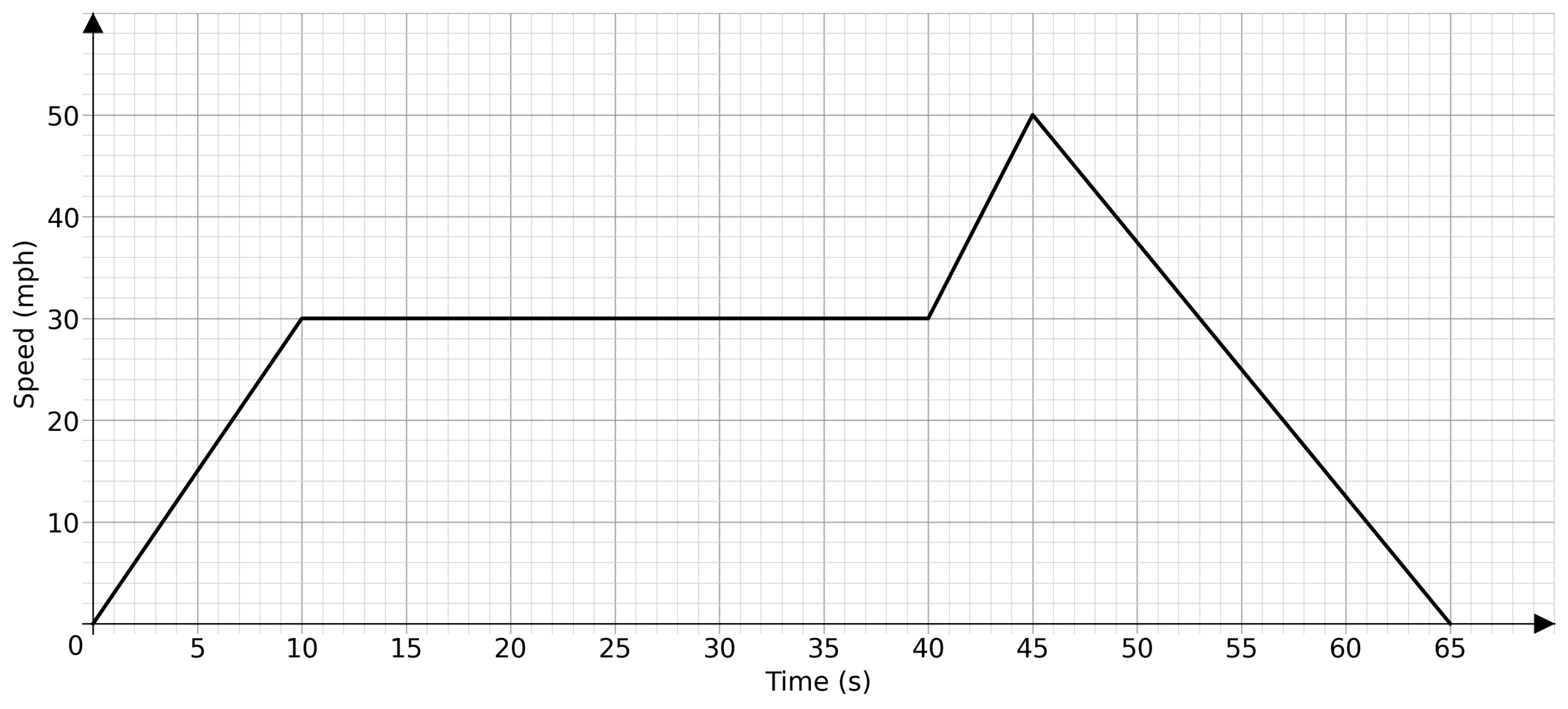Level 4-5GCSEEdexcel iGCSE

## Speed-Time Graphs Example Questions

a) The total distance travelled is the area under the graph. It will then be easier to break the graph into sections with easy to work out sections.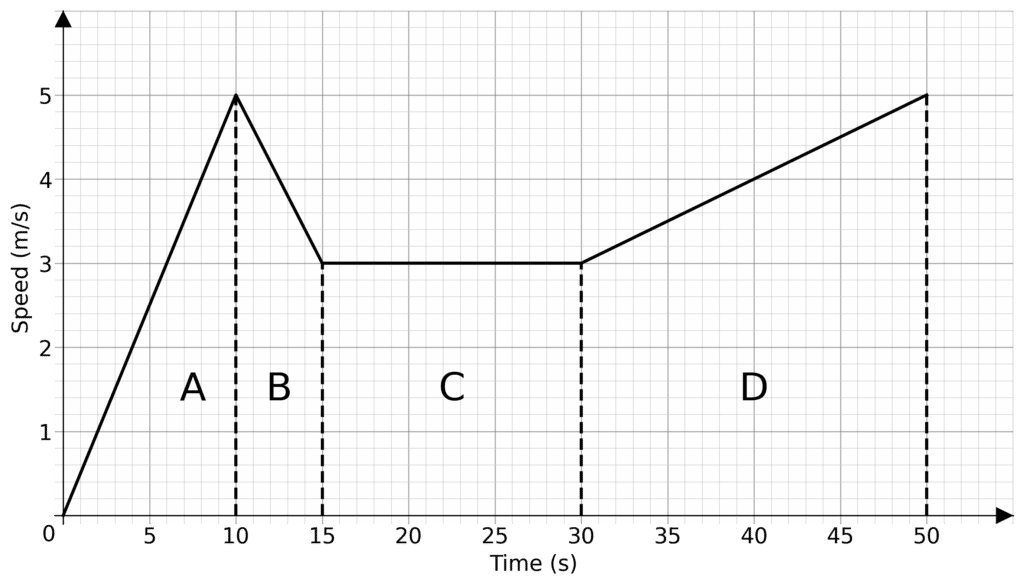Here, sections $A$ is a triangle, $B$ and $D$ are trapeziums and $C$ is a rectangle.

$\text{Area }A = \dfrac{1}{2}\times 10 \times 5 = 25$

$\text{Area }B = \dfrac{5+3}{2}\times 5 = 20$

$\text{Area }C = 15 \times 3 = 45$

$\text{Area }D = \dfrac{3+5}{2}\times 20 = 80$

So the total area is

$\text{Total Area} = 25+20+45+80=170$

So the distance travelled is $170 \text{ m}$

b) The acceleration is the gradient. Between $0$ and $10$ seconds it has a constant constant gradient as it is a straight line. So using the points $(0,0)$ and $(10,5)$ we get a gradient of

$\text{Gradient} = \dfrac{5-0}{10-0} = 0.5$

So the acceleration is $0.5 \text{ ms}^{-2}$

We begin the journey at $0$ seconds and $0 \text{ m/s}$. We can then draw a straight line from $(0,0)$ to $(15, 20)$ as the train accelerates. Then we may draw a flat line from $(15,20)$ to $(60, 20)$ as the train travels at a constant speed.

We need to work out how long the train takes to come to a stop. If it was increasing in speed at $1.25 \text{ ms}^{-2}$ to $20\text{ m/s}$ we would have

$1.25 t = 20$

$t = \dfrac{20}{1.25} = 16 \text{ s}$

Therefor it would take $16$ seconds for the train to decelerate from $20\text{ m/s}$ to $0\text{ m/s}$ at the same rate.

So we may draw a straight line from $(60, 20)$ to $(76, 0)$, giving us a graph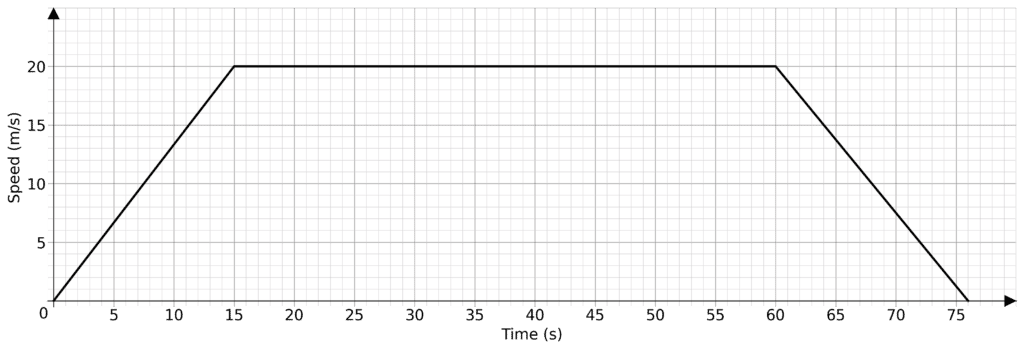a) For section A: The acceleration is the gradient. Given points $(0,0)$ and $(15, 3)$

$\text{Gradient} = \dfrac{3-0}{15-0} = 0.2$

Acceleration is $0.2 \text{ ms}^2$

For section B: The acceleration is the gradient. Given points $(15,3)$ and $(20, 5)$

$\text{Gradient} = \dfrac{5-3}{20-15} = 0.4$

Acceleration is $0.4 \text{ ms}^2$

For section C: As this is a flat line the acceleration is $0 \text{ ms}^2$

For section D: The acceleration is the gradient. Given points $(40,5)$ and $(50, 0)$

$\text{Gradient} = \dfrac{0-5}{50-40} = -0.5$

The deceleration is $0.5 \text{ ms}^2$

b) The total distance travelled is the area under the graph.

$\text{Area }A = \dfrac{1}{2}\times 15 \times 2 = 22.5$

$\text{Area }B = \dfrac{5+3}{2}\times 5 = 20$

$\text{Area }C = 20 \times 5 = 100$

$\text{Area }D = \dfrac{1}{2}\times 5 \times 10 = 25$

So the total area is

$\text{Total Area} = 22.5+20+100+25= 167.5$

So the distance travelled is $167.5 \text{ m}$

## You May Also Like...### MME Learning Portal

Online exams, practice questions and revision videos for every GCSE level 9-1 topic! No fees, no trial period, just totally free access to the UK’s best GCSE maths revision platform.

£0.00